# *【一天一题—Day55】1608. 特殊数组的特征值

## 二、题目

1608. 特殊数组的特征值

``````给你一个非负整数数组 nums 。如果存在一个数 x ，使得 nums 中恰好有 x 个元素 大于或者等于 x ，那么就称 nums 是一个 特殊数组 ，而 x 是该数组的 特征值 。

``````

``````输入：nums = [3,5]

``````

``````输入：nums = [0,0]

x 不能取更大的值，因为 nums 中只有两个元素。
``````

``````输入：nums = [0,4,3,0,4]

``````

``````输入：nums = [3,6,7,7,0]

``````

• 1 <= nums.length <= 100
• 0 <= nums[i] <= 1000

# *【一天一题—Day54】857. 雇佣 K 名工人的最低成本

## 二、题目

857. 雇佣 K 名工人的最低成本

``````有 n 名工人。 给定两个数组 quality 和 wage ，其中，quality[i] 表示第 i 名工人的工作质量，其最低期望工资为 wage[i] 。

``````

``````输入： quality = [10,20,5], wage = [70,50,30], k = 2

``````

``````输入： quality = [3,1,10,10,1], wage = [4,8,2,2,7], k = 3

``````

• n == quality.length == wage.length
• 1 <= k <= n <= 104
• 1 <= quality[i], wage[i] <= 104

# *【一天一题—Day53】669. 修剪二叉搜索树

## 二、题目

``````给你二叉搜索树的根节点 root ，同时给定最小边界low 和最大边界 high。通过修剪二叉搜索树，使得所有节点的值在[low, high]中。修剪树 不应该 改变保留在树中的元素的相对结构 (即，如果没有被移除，原有的父代子代关系都应当保留)。 可以证明，存在 唯一的答案 。

``````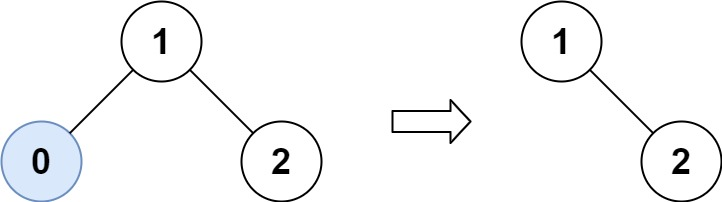``````输入：root = [1,0,2], low = 1, high = 2

``````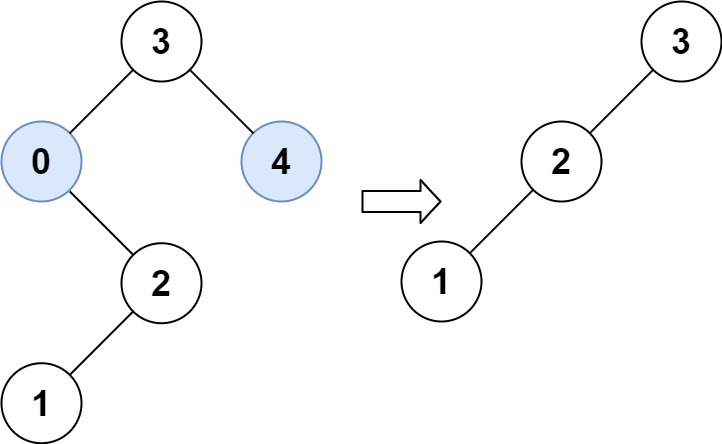``````输入：root = [3,0,4,null,2,null,null,1], low = 1, high = 3

``````

• 树中节点数在范围 [1, 104] 内
• 0 <= Node.val <= 104
• 树中每个节点的值都是 唯一 的
• 题目数据保证输入是一棵有效的二叉搜索树
• 0 <= low <= high <= 104

# *【一天一题—Day52】1598. 文件夹操作日志搜集器

## 二、题目

``````每当用户执行变更文件夹操作时，LeetCode 文件系统都会保存一条日志记录。

"../" ：移动到当前文件夹的父文件夹。如果已经在主文件夹下，则 继续停留在当前文件夹 。
"./" ：继续停留在当前文件夹。
"x/" ：移动到名为 x 的子文件夹中。题目数据 保证总是存在文件夹 x 。
``````

``````文件系统启动时位于主文件夹，然后执行 logs 中的操作。

``````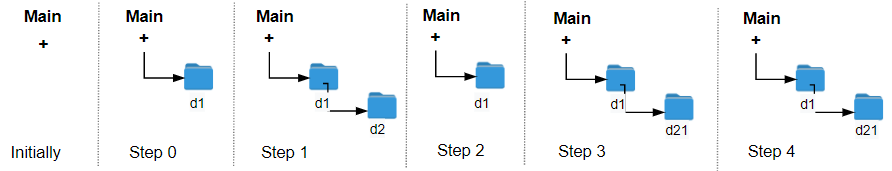``````输入：logs = ["d1/","d2/","../","d21/","./"]

````````````输入：logs = ["d1/","d2/","./","d3/","../","d31/"]

``````

``````输入：logs = ["d1/","../","../","../"]

``````

• 1 <= logs.length <= 103
• 2 <= logs[i].length <= 10
• logs[i] 包含小写英文字母，数字，’.’ 和 ‘/‘
• logs[i] 符合语句中描述的格式
• 文件夹名称由小写英文字母和数字组成

# *【一天一题—Day51】667. 优美的排列 II

## 二、题目

``````给你两个整数 n 和 k ，请你构造一个答案列表 answer ，该列表应当包含从 1 到 n 的 n 个不同正整数，并同时满足下述条件：

``````

``````输入：n = 3, k = 1

``````

``````输入：n = 3, k = 2

``````

• 1 <= k < n <= 104

## 三、解答

• @Kwbdlhisq
初看没思路，随便找个大点的用例一下就能找到规律。 [1, 1+k, 2, 1+k-1, 3, 1+k-2, 4…..k+2, k+3…n]

# *【一天一题—Day50】1592. 重新排列单词间的空格

## 二、题目

``````给你一个字符串 text ，该字符串由若干被空格包围的单词组成。每个单词由一个或者多个小写英文字母组成，并且两个单词之间至少存在一个空格。题目测试用例保证 text 至少包含一个单词 。

``````

``````输入：text = "  this   is  a sentence "

``````

``````输入：text = " practice   makes   perfect"

``````

``````输入：text = "hello   world"

``````

``````输入：text = "  walks  udp package   into  bar a"

``````

``````输入：text = "a"

``````

• 1 <= text.length <= 100
• text 由小写英文字母和 ‘ ‘ 组成
• text 中至少包含一个单词

# *【一天一题—Day49】828. 统计子串中的唯一字符

## 二、题目

``````我们定义了一个函数 countUniqueChars(s) 来统计字符串 s 中的唯一字符，并返回唯一字符的个数。

``````

``````输入: s = "ABC"

其中，每一个子串都由独特字符构成。
所以其长度总和为：1 + 1 + 1 + 2 + 2 + 3 = 10
``````

``````输入: s = "ABA"

``````

``````输入：s = "LEETCODE"

``````

• 1 <= s.length <= 10^5
• s 只包含大写英文字符

# 【一天一题—Day48】652. 寻找重复的子树

## 二、题目

``````给你一棵二叉树的根节点 root ，返回所有 重复的子树 。

``````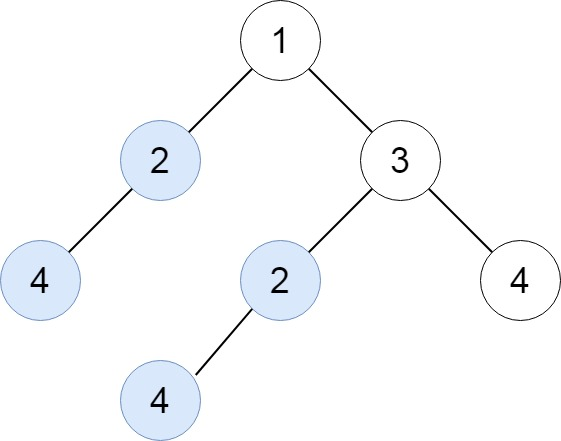``````输入：root = [1,2,3,4,null,2,4,null,null,4]

``````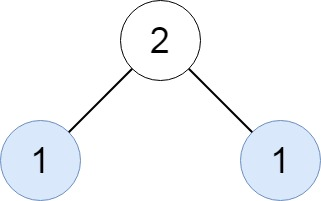``````输入：root = [2,1,1]

``````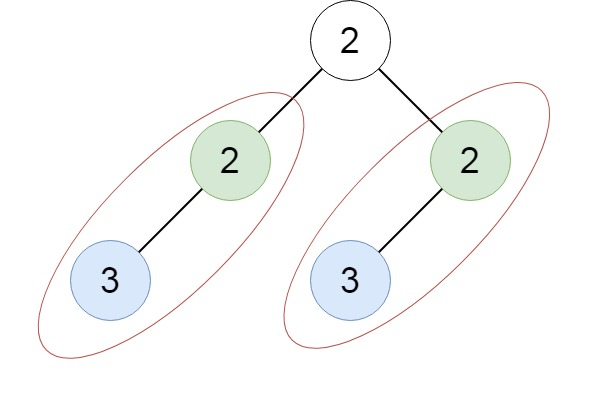``````输入：root = [2,2,2,3,null,3,null]

``````

• 树中的结点数在 [1, 5000] 范围内。
• -200 <= Node.val <= 200

# 【一天一题—Day47】1582. 二进制矩阵中的特殊位置

## 二、题目

``````给你一个大小为 rows x cols 的矩阵 mat，其中 mat[i][j] 是 0 或 1，请返回 矩阵 mat 中特殊位置的数目 。

``````

``````输入：mat = [[1,0,0],
[0,0,1],
[1,0,0]]

``````

``````输入：mat = [[1,0,0],
[0,1,0],
[0,0,1]]

``````

``````输入：mat = [[0,0,0,1],
[1,0,0,0],
[0,1,1,0],
[0,0,0,0]]

``````

``````输入：mat = [[0,0,0,0,0],
[1,0,0,0,0],
[0,1,0,0,0],
[0,0,1,0,0],
[0,0,0,1,1]]

``````

• rows == mat.length
• cols == mat[i].length
• 1 <= rows, cols <= 100
• mat[i][j] 是 0 或 1

# 【一天一题—Day46】646. 最长数对链

## 二、题目

``````给出 n 个数对。 在每一个数对中，第一个数字总是比第二个数字小。

``````

``````输入：[[1,2], [2,3], [3,4]]

``````

• 给出数对的个数在 [1, 1000] 范围内。

# 【一天一题—Day45】687. 最长同值路径

## 二、题目

``````给定一个二叉树的 root ，返回 最长的路径的长度 ，这个路径中的 每个节点具有相同值 。 这条路径可以经过也可以不经过根节点。

``````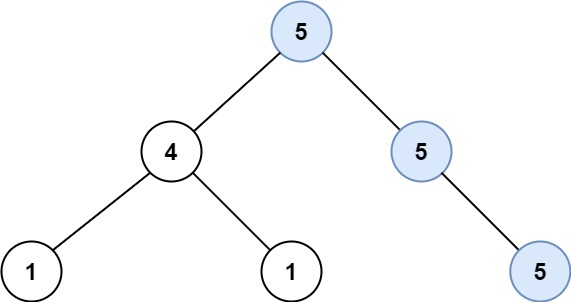``````输入：root = [5,4,5,1,1,5]

``````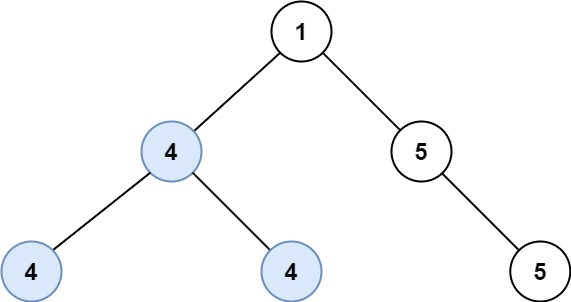``````输入：root = [1,4,5,4,4,5]

``````

• 树的节点数的范围是 [0, 104]
• -1000 <= Node.val <= 1000
• 树的深度将不超过 1000

# 【一天一题—Day44】1475. 商品折扣后的最终价格

## 一、前景提要## 二、题目

``````给你一个数组 prices ，其中 prices[i] 是商店里第 i 件商品的价格。

``````

``````输入：prices = [8,4,6,2,3]

``````

``````输入：prices = [1,2,3,4,5]

``````

``````输入：prices = [10,1,1,6]

``````

• 1 <= prices.length <= 500
• 1 <= prices[i] <= 10^3

# 【一天一题—Day43】998. 最大二叉树 II

## 二、题目

``````最大树 定义：一棵树，并满足：其中每个节点的值都大于其子树中的任何其他值。

root 的左子树将被构建为 Construct([a, a, ..., a[i - 1]]) 。
root 的右子树将被构建为 Construct([a[i + 1], a[i + 2], ..., a[a.length - 1]]) 。

``````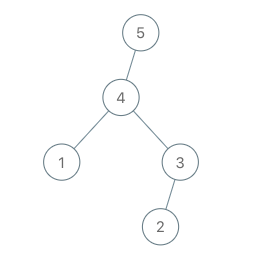``````输入：root = [4,1,3,null,null,2], val = 5

``````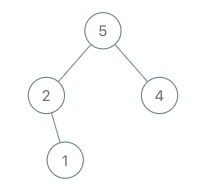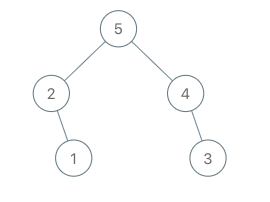``````输入：root = [5,2,4,null,1], val = 3

``````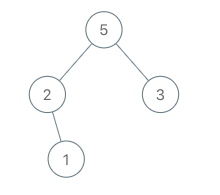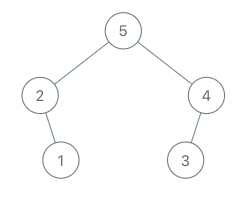``````输入：root = [5,2,3,null,1], val = 4

``````

• 树中节点数目在范围 [1, 100] 内
• 1 <= Node.val <= 100
• 树中的所有值 互不相同
• 1 <= val <= 100

# *【一天一题—Day42】946. 验证栈序列

## 二、题目

``````给定 pushed 和 popped 两个序列，每个序列中的 值都不重复，只有当它们可能是在最初空栈上进行的推入 push 和弹出 pop 操作序列的结果时，返回 true；否则，返回 false 。
``````

``````输入：pushed = [1,2,3,4,5], popped = [4,5,3,2,1]

push(1), push(2), push(3), push(4), pop() -> 4,
push(5), pop() -> 5, pop() -> 3, pop() -> 2, pop() -> 1
``````

``````输入：pushed = [1,2,3,4,5], popped = [4,3,5,1,2]

``````

• 1 <= pushed.length <= 1000
• 0 <= pushed[i] <= 1000
• pushed 的所有元素 互不相同
• popped.length == pushed.length
• popped 是 pushed 的一个排列

# 【一天一题—Day41】793. 阶乘函数后 K 个零

## 二、题目

`````` f(x) 是 x! 末尾是 0 的数量。回想一下 x! = 1 * 2 * 3 * ... * x，且 0! = 1 。

``````

``````输入：k = 0

``````

``````输入：k = 5

``````

``````输入: k = 3

``````

• 0 <= k <= 109

# 【一天一题—Day40】1470. 重新排列数组

## 二、题目

``````给你一个数组 nums ，数组中有 2n 个元素，按 [x1,x2,...,xn,y1,y2,...,yn] 的格式排列。

``````

``````输入：nums = [2,5,1,3,4,7], n = 3

``````

``````输入：nums = [1,2,3,4,4,3,2,1], n = 4

``````

``````输入：nums = [1,1,2,2], n = 2

``````

• 1 <= n <= 500
• nums.length == 2n
• 1 <= nums[i] <= 10^3

# 【一天一题—Day39】1470. 重新排列数组

## 二、题目

``````给你一个数组 nums ，数组中有 2n 个元素，按 [x1,x2,...,xn,y1,y2,...,yn] 的格式排列。

``````

``````输入：nums = [2,5,1,3,4,7], n = 3

``````

``````输入：nums = [1,2,3,4,4,3,2,1], n = 4

``````

``````输入：nums = [1,1,2,2], n = 2

``````

• 1 <= n <= 500
• nums.length == 2n
• 1 <= nums[i] <= 10^3

# 【一天一题—Day38】662. 二叉树最大宽度

## 二、题目

``````给你一棵二叉树的根节点 root ，返回树的 最大宽度 。

``````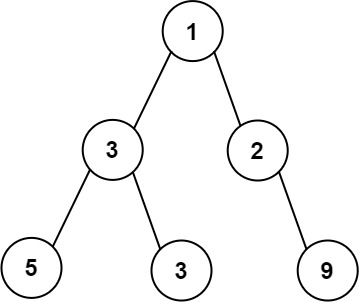``````输入：root = [1,3,2,5,3,null,9]

``````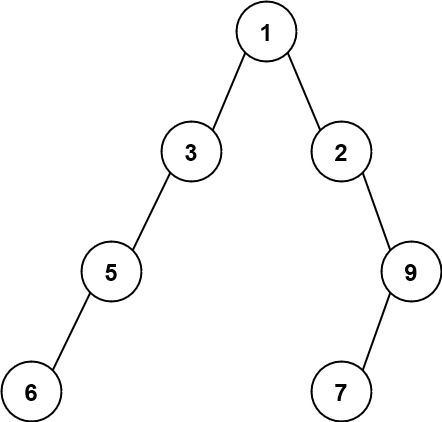``````输入：root = [1,3,2,5,null,null,9,6,null,7]

``````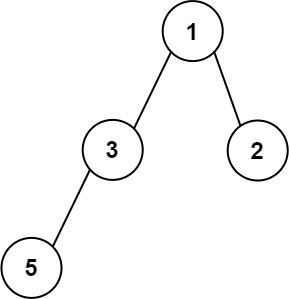``````输入：root = [1,3,2,5]

``````

• 树中节点的数目范围是 [1, 3000]
• -100 <= Node.val <= 100

# 【一天一题—Day37】1464. 数组中两元素的最大乘积

## 二、题目

``````给你一个整数数组 nums，请你选择数组的两个不同下标 i 和 j，使 	(nums[i]-1)*(nums[j]-1) 取得最大值。

``````

``````输入：nums = [3,4,5,2]

``````

``````输入：nums = [1,5,4,5]

``````

``````输入：nums = [3,7]

``````

• 2 <= nums.length <= 500
• 1 <= nums[i] <= 10^3

# 【一天一题—Day36】658. 找到 K 个最接近的元素

## 二、题目

``````给定一个 排序好 的数组 arr ，两个整数 k 和 x ，从数组中找到最靠近 x（两数之差最小）的 k 个数。返回的结果必须要是按升序排好的。

|a - x| < |b - x| 或者
|a - x| == |b - x| 且 a < b
``````

``````输入：arr = [1,2,3,4,5], k = 4, x = 3

``````

``````输入：arr = [1,2,3,4,5], k = 4, x = -1

``````

• 1 <= k <= arr.length
• 1 <= arr.length <= 104
• arr 按 升序 排列
• -104 <= arr[i], x <= 104

# 【一天一题—Day35】1460. 通过翻转子数组使两个数组相等

## 二、题目

``````给你两个长度相同的整数数组 target 和 arr 。每一步中，你可以选择 arr 的任意 非空子数组 并将它翻转。你可以执行此过程任意次。

``````

``````输入：target = [1,2,3,4], arr = [2,4,1,3]

1- 翻转子数组 [2,4,1] ，arr 变成 [1,4,2,3]
2- 翻转子数组 [4,2] ，arr 变成 [1,2,4,3]
3- 翻转子数组 [4,3] ，arr 变成 [1,2,3,4]

``````

``````输入：target = , arr = 

``````

``````输入：target = [3,7,9], arr = [3,7,11]

``````

• target.length == arr.length
• 1 <= target.length <= 1000
• 1 <= target[i] <= 1000
• 1 <= arr[i] <= 1000

# *【一天一题—Day33】655. 输出二叉树

## 二、题目

``````给你一棵二叉树的根节点 root ，请你构造一个下标从 0 开始、大小为 m x n 的字符串矩阵 res ，用以表示树的 格式化布局 。构造此格式化布局矩阵需要遵循以下规则：

- 树的 高度 为 height ，矩阵的行数 m 应该等于 height + 1 。
- 矩阵的列数 n 应该等于 2height+1 - 1 。
- 根节点 需要放置在 顶行 的 正中间 ，对应位置为 res[(n-1)/2] 。
- 对于放置在矩阵中的每个节点，设对应位置为 res[r][c] ，将其左子节点放置在 res[r+1][c-2height-r-1] ，右子节点放置在 res[r+1][c+2height-r-1] 。
- 继续这一过程，直到树中的所有节点都妥善放置。
- 任意空单元格都应该包含空字符串 "" 。

``````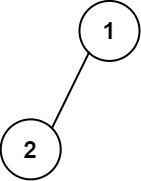``````输入：root = [1,2]

[["","1",""],
["2","",""]]
``````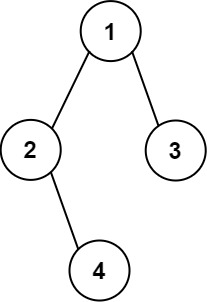``````输入：root = [1,2,3,null,4]

[["","","","1","","",""],
["","2","","","","3",""],
["","","4","","","",""]]
``````

• 树中节点数在范围 [1, 210] 内
• -99 <= Node.val <= 99
• 树的深度在范围 [1, 10] 内

# 【一天一题—Day32】1455. 检查单词是否为句中其他单词的前缀

## 二、题目

1455.检查单词是否为句中其他单词的前缀

``````给你一个字符串 sentence 作为句子并指定检索词为 searchWord ，其中句子由若干用 单个空格 分隔的单词组成。请你检查检索词 searchWord 是否为句子 sentence 中任意单词的前缀。

``````

``````输入：sentence = "i love eating burger", searchWord = "burg"

``````

``````输入：sentence = "this problem is an easy problem", searchWord = "pro"

``````

``````输入：sentence = "i am tired", searchWord = "you"

``````

• 1 <= sentence.length <= 100
• 1 <= searchWord.length <= 10
• sentence 由小写英文字母和空格组成。
• searchWord 由小写英文字母组成。

# 【一天一题—Day31】654. 最大二叉树

## 二、题目

``````给定一个不重复的整数数组 nums 。 最大二叉树 可以用下面的算法从 nums 递归地构建:

``````

``````输入：nums = [3,2,1,6,0,5]

- [3,2,1,6,0,5] 中的最大值是 6 ，左边部分是 [3,2,1] ，右边部分是 [0,5] 。
- [3,2,1] 中的最大值是 3 ，左边部分是 [] ，右边部分是 [2,1] 。
- 空数组，无子节点。
- [2,1] 中的最大值是 2 ，左边部分是 [] ，右边部分是  。
- 空数组，无子节点。
- 只有一个元素，所以子节点是一个值为 1 的节点。
- [0,5] 中的最大值是 5 ，左边部分是  ，右边部分是 [] 。
- 只有一个元素，所以子节点是一个值为 0 的节点。
- 空数组，无子节点。
``````

``````输入：nums = [3,2,1]

``````

• 1 <= nums.length <= 1000
• 0 <= nums[i] <= 1000
• nums 中的所有整数 互不相同

# 【一天一题—Day30】1450. 在既定时间做作业的学生人数

## 二、题目

``````给你两个整数数组 startTime（开始时间）和 endTime（结束时间），并指定一个整数 queryTime 作为查询时间。

``````

``````输入：startTime = [1,2,3], endTime = [3,2,7], queryTime = 4

``````

``````输入：startTime = , endTime = , queryTime = 4

``````

``````输入：startTime = , endTime = , queryTime = 5

``````

``````输入：startTime = [1,1,1,1], endTime = [1,3,2,4], queryTime = 7

``````

``````输入：startTime = [9,8,7,6,5,4,3,2,1], endTime = [10,10,10,10,10,10,10,10,10], queryTime = 5

``````

• startTime.length == endTime.length
• 1 <= startTime.length <= 100
• 1 <= startTime[i] <= endTime[i] <= 1000
• 1 <= queryTime <= 1000

# *【一天一题—Day29】1224. 最大相等频率

## 二、题目

``````给你一个正整数数组 nums，请你帮忙从该数组中找出能满足下面要求的 最长 前缀，并返回该前缀的长度：

``````

``````输入：nums = [2,2,1,1,5,3,3,5]

``````

``````输入：nums = [1,1,1,2,2,2,3,3,3,4,4,4,5]

``````

• 2 <= nums.length <= 105
• 1 <= nums[i] <= 105

# 【一天一题—Day28】1302. 层数最深叶子节点的和

## 二、题目

``````给你一棵二叉树的根节点 root ，请你返回 层数最深的叶子节点的和 。
``````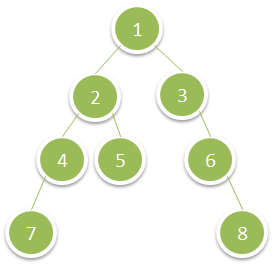``````输入：root = [6,7,8,2,7,1,3,9,null,1,4,null,null,null,5]

``````

• 树中节点数目在范围 [1, 104] 之间。
• 1 <= Node.val <= 100

## 三、解答

### 1，非官方解法

dfs，最开始我只写了简单的dfs但是有问题，题目说的最深层的我以为每个子树最深加起来就行，后来才发现是最深层的和，不是最深层的不算

# 【一天一题—Day27】1656. 设计有序流

## 二、题目

``````有 n 个 (id, value) 对，其中 id 是 1 到 n 之间的一个整数，value 是一个字符串。不存在 id 相同的两个 (id, value) 对。

- OrderedStream(int n) 构造一个能接收 n 个值的流，并将当前指针 ptr 设为 1 。
- String[] insert(int id, String value) 向流中存储新的 (id, value) 对。存储后：
-- 如果流存储有 id = ptr 的 (id, value) 对，则找出从 id = ptr 开始的 最长 id 连续递增序列 ，并 按顺序 返回与这些 id 关联的值的列表。然后，将 ptr 更新为最后那个  id + 1 。
-- 否则，返回一个空列表。
``````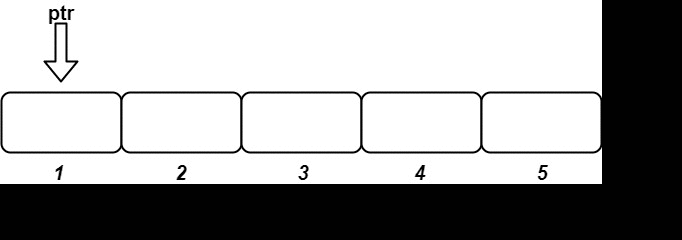``````输入
["OrderedStream", "insert", "insert", "insert", "insert", "insert"]
[, [3, "ccccc"], [1, "aaaaa"], [2, "bbbbb"], [5, "eeeee"], [4, "ddddd"]]

[null, [], ["aaaaa"], ["bbbbb", "ccccc"], [], ["ddddd", "eeeee"]]

OrderedStream os= new OrderedStream(5);
os.insert(3, "ccccc"); // 插入 (3, "ccccc")，返回 []
os.insert(1, "aaaaa"); // 插入 (1, "aaaaa")，返回 ["aaaaa"]
os.insert(2, "bbbbb"); // 插入 (2, "bbbbb")，返回 ["bbbbb", "ccccc"]
os.insert(5, "eeeee"); // 插入 (5, "eeeee")，返回 []
os.insert(4, "ddddd"); // 插入 (4, "ddddd")，返回 ["ddddd", "eeeee"]
``````

• 1 <= n <= 1000
• 1 <= id <= n
• value.length == 5
• value 仅由小写字母组成
• 每次调用 insert 都会使用一个唯一的 id
• 恰好调用 n 次 insert

# *【一天一题—Day26】641. 设计循环双端队列

## 二、题目

``````设计实现双端队列。

- MyCircularDeque(int k) ：构造函数,双端队列最大为 k 。
- boolean insertFront()：将一个元素添加到双端队列头部。 如果操作成功返回 true ，否则返回 false 。
- boolean insertLast() ：将一个元素添加到双端队列尾部。如果操作成功返回 true ，否则返回 false 。
- boolean deleteFront() ：从双端队列头部删除一个元素。 如果操作成功返回 true ，否则返回 false 。
- boolean deleteLast() ：从双端队列尾部删除一个元素。如果操作成功返回 true ，否则返回 false 。
- int getFront() )：从双端队列头部获得一个元素。如果双端队列为空，返回 -1 。
- int getRear() ：获得双端队列的最后一个元素。 如果双端队列为空，返回 -1 。
- boolean isEmpty() ：若双端队列为空，则返回 true ，否则返回 false  。
- boolean isFull() ：若双端队列满了，则返回 true ，否则返回 false 。
``````

``````输入
["MyCircularDeque", "insertLast", "insertLast", "insertFront", "insertFront", "getRear", "isFull", "deleteLast", "insertFront", "getFront"]
[, , , , , [], [], [], , []]

[null, true, true, true, false, 2, true, true, true, 4]

MyCircularDeque circularDeque = new MycircularDeque(3); // 设置容量大小为3
circularDeque.insertLast(1);			        // 返回 true
circularDeque.insertLast(2);			        // 返回 true
circularDeque.insertFront(3);			        // 返回 true
circularDeque.insertFront(4);			        // 已经满了，返回 false
circularDeque.getRear();  				// 返回 2
circularDeque.isFull();				        // 返回 true
circularDeque.deleteLast();			        // 返回 true
circularDeque.insertFront(4);			        // 返回 true
circularDeque.getFront();				// 返回 4
``````

• 1 <= k <= 1000
• 0 <= value <= 1000
• insertFront, insertLast, deleteFront, deleteLast, getFront, getRear, isEmpty, isFull 调用次数不大于 2000 次

# 【一天一题—Day25】1422. 分割字符串的最大得分

## 二、题目

``````给你一个由若干 0 和 1 组成的字符串 s ，请你计算并返回将该字符串分割成两个 非空 子字符串（即 左 子字符串和 右 子字符串）所能获得的最大得分。

「分割字符串的得分」为 左 子字符串中 0 的数量加上 右 子字符串中 1 的数量。
``````

``````输入：s = "011101"

``````

``````输入：s = "00111"

``````

``````输入：s = "1111"

``````

• 2 <= s.length <= 500
• 字符串 s 仅由字符 ‘0’ 和 ‘1’ 组成。

## 三、解答

### 1，非官方解法

``````执行用时：1880 ms, 在所有 Java 提交中击败了5.74%的用户

``````

# 【一天一题—Day24】1282. 用户分组

## 二、题目

``````有 n 个人被分成数量未知的组。每个人都被标记为一个从 0 到 n - 1 的唯一ID 。

``````

``````输入：groupSizes = [3,3,3,3,3,1,3]

``````

``````输入：groupSizes = [2,1,3,3,3,2]

``````

• groupSizes.length == n
• 1 <= n <= 500
• 1 <= groupSizes[i] <= n

# 【一天一题—Day23】1417. 重新格式化字符串

## 二、题目

``````给你一个混合了数字和字母的字符串 s，其中的字母均为小写英文字母。

``````

``````输入：s = "a0b1c2"

``````

``````输入：s = "leetcode"

``````

``````输入：s = "1229857369"

``````

``````输入：s = "covid2019"

``````

``````输入：s = "ab123"

``````

• 1 <= s.length <= 500
• s 仅由小写英文字母和/或数字组成。

# 【一天一题—Day22】640. 求解方程

## 二、题目

``````求解一个给定的方程，将x以字符串 "x=#value" 的形式返回。该方程仅包含 '+' ， '-' 操作，变量 x 和其对应系数。

``````

``````输入：nums = [-3,2,-3,4,2]

累加求和
startValue = 4 | startValue = 5 | nums
(4 -3 ) = 1  | (5 -3 ) = 2    |  -3
(1 +2 ) = 3  | (2 +2 ) = 4    |   2
(3 -3 ) = 0  | (4 -3 ) = 1    |  -3
(0 +4 ) = 4  | (1 +4 ) = 5    |   4
(4 +2 ) = 6  | (5 +2 ) = 7    |   2
``````

``````输入：nums = [1,2]

``````

``````输入：nums = [1,-2,-3]

``````

• 1 <= nums.length <= 100
• -100 <= nums[i] <= 100

buhui

# 【一天一题—Day21】1413. 逐步求和得到正数的最小值

## 二、题目

``````给你一个整数数组 nums 。你可以选定任意的 正数 startValue 作为初始值。

``````

``````输入：nums = [-3,2,-3,4,2]

累加求和
startValue = 4 | startValue = 5 | nums
(4 -3 ) = 1  | (5 -3 ) = 2    |  -3
(1 +2 ) = 3  | (2 +2 ) = 4    |   2
(3 -3 ) = 0  | (4 -3 ) = 1    |  -3
(0 +4 ) = 4  | (1 +4 ) = 5    |   4
(4 +2 ) = 6  | (5 +2 ) = 7    |   2
``````

``````输入：nums = [1,2]

``````

``````输入：nums = [1,-2,-3]

``````

• 1 <= nums.length <= 100
• -100 <= nums[i] <= 100

# *【一天一题—Day20】761. 特殊的二进制序列

## 二、题目

``````特殊的二进制序列是具有以下两个性质的二进制序列：

- 0 的数量与 1 的数量相等。
- 二进制序列的每一个前缀码中 1 的数量要大于等于 0 的数量。
-

``````

``````输入：words = ["mass","as","hero","superhero"]

["hero","as"] 也是有效的答案。
``````

``````输入: S = "11011000"

``````

• S 的长度不超过 50。
• S 保证为一个满足上述定义的特殊 的二进制序列。

• 1
• 2

# *【一天一题—Day19】636. 函数的独占时间

## 二、题目

``````有一个 单线程 CPU 正在运行一个含有 n 道函数的程序。每道函数都有一个位于  0 和 n-1 之间的唯一标识符。

``````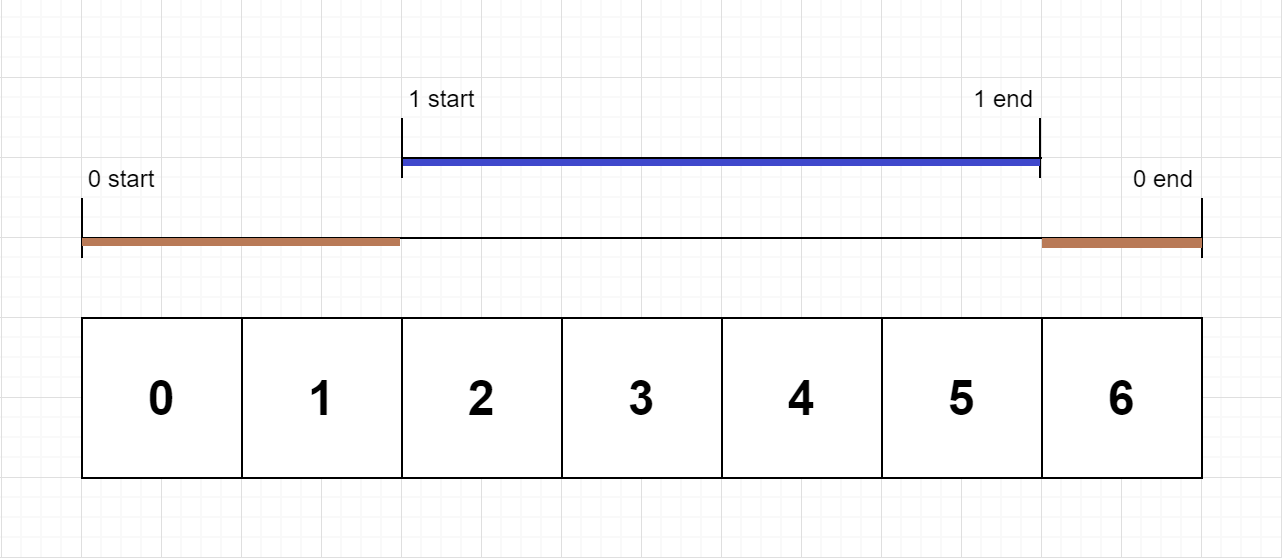``````输入：n = 2, logs = ["0:start:0","1:start:2","1:end:5","0:end:6"]

``````

``````输入：n = 1, logs = ["0:start:0","0:start:2","0:end:5","0:start:6","0:end:6","0:end:7"]

``````

``````输入：n = 2, logs = ["0:start:0","0:start:2","0:end:5","1:start:6","1:end:6","0:end:7"]

``````

``````输入：n = 2, logs = ["0:start:0","0:start:2","0:end:5","1:start:7","1:end:7","0:end:8"]

``````

``````输入：n = 1, logs = ["0:start:0","0:end:0"]

``````

• 1 <= n <= 100
• 1 <= logs.length <= 500
• 0 <= function_id < n
• 0 <= timestamp <= 109
• 两个开始事件不会在同一时间戳发生
• 两个结束事件不会在同一时间戳发生
• 每道函数都有一个对应 “start” 日志的 “end” 日志

# 【一天一题—Day18】1408. 数组中的字符串匹配

## 二、题目

``````给你一个字符串数组 words ，数组中的每个字符串都可以看作是一个单词。请你按 任意 顺序返回 words 中是其他单词的子字符串的所有单词。

``````

``````输入：words = ["mass","as","hero","superhero"]

["hero","as"] 也是有效的答案。
``````

``````输入：words = ["leetcode","et","code"]

``````

``````输入：words = ["blue","green","bu"]

``````

• 1 <= words.length <= 100
• 1 <= words[i].length <= 30
• words[i] 仅包含小写英文字母。
• 题目数据 保证 每个 words[i] 都是独一无二的。

# 【一天一题—Day17】623. 在二叉树中增加一行

## 二、题目

``````给定一个二叉树的根 root 和两个整数 val 和 depth ，在给定的深度 depth 处添加一个值为 val 的节点行。

- 给定整数 depth，对于深度为 depth - 1 的每个非空树节点 cur ，创建两个值为 val 的树节点作为 cur 的左子树根和右子树根。
- cur 原来的左子树应该是新的左子树根的左子树。
- cur 原来的右子树应该是新的右子树根的右子树。
- 如果 depth == 1 意味着 depth - 1 根本没有深度，那么创建一个树节点，值 val 作为整个原始树的新根，而原始树就是新根的左子树。
``````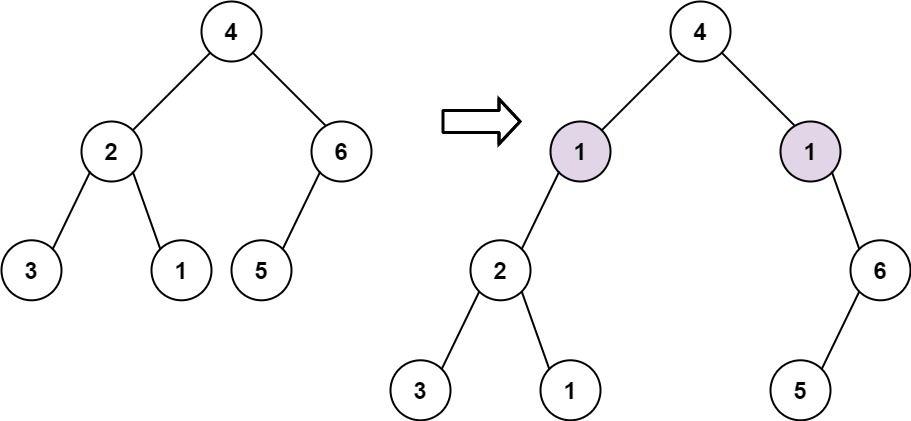``````输入: root = [4,2,6,3,1,5], val = 1, depth = 2

``````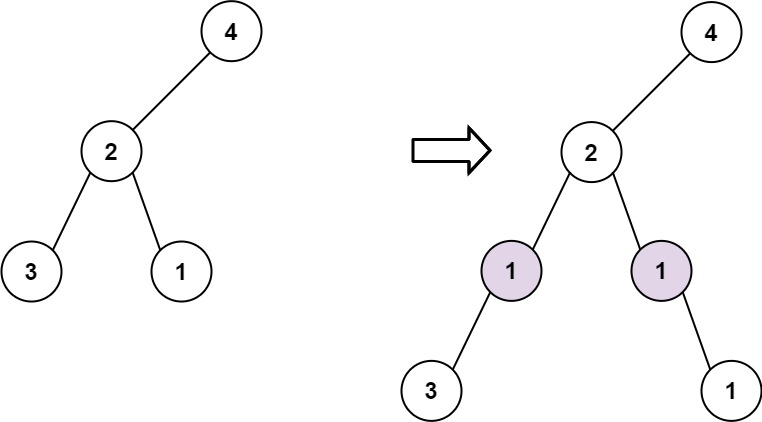``````输入: root = [4,2,null,3,1], val = 1, depth = 3

``````

• 节点数在 [1, 104] 范围内
• 树的深度在 [1, 104]范围内
• -100 <= Node.val <= 100
• -105 <= val <= 105
• 1 <= depth <= the depth of tree + 1

## 三、解答

### 1，非官方解法

DFS，递归往下层搜索，但是优雅版

DFS

# 【一天一题—Day16】1403. 非递增顺序的最小子序列

## 二、题目

``````给你一个数组 nums，请你从中抽取一个子序列，满足该子序列的元素之和 严格 大于未包含在该子序列中的各元素之和。

``````

``````输入：nums = [4,3,10,9,8]

``````

``````输入：nums = [4,4,7,6,7]

``````

``````输入：nums = 

``````

• 1 <= nums.length <= 500
• 1 <= nums[i] <= 100

# 【一天一题—Day15】899. 有序队列

## 一、前景提要

java多线程何时我才能认真学完啊！……

## 二、题目

``````给定一个字符串 s 和一个整数 k 。你可以从 s 的前 k 个字母中选择一个，并把它加到字符串的末尾。

``````

``````输入：s = "cba", k = 1

``````

``````输入：s = "baaca", k = 3

``````

• 1 <= k <= S.length <= 1000
• s 只由小写字母组成。

## 三、解答

### 1，非官方解法

Python/Java/TypeScript/Go 脑筋急转弯

# 【一天一题—Day14】622. 设计循环队列

## 一、前景提要

java多线程何时我才能认真学完啊！……

## 二、题目

``````设计你的循环队列实现。 循环队列是一种线性数据结构，其操作表现基于 FIFO（先进先出）原则并且队尾被连接在队首之后以形成一个循环。它也被称为“环形缓冲器”。

MyCircularQueue(k): 构造器，设置队列长度为 k 。
Front: 从队首获取元素。如果队列为空，返回 -1 。
Rear: 获取队尾元素。如果队列为空，返回 -1 。
enQueue(value): 向循环队列插入一个元素。如果成功插入则返回真。
deQueue(): 从循环队列中删除一个元素。如果成功删除则返回真。
isEmpty(): 检查循环队列是否为空。
isFull(): 检查循环队列是否已满。
``````

``````MyCircularQueue circularQueue = new MyCircularQueue(3); // 设置长度为 3
circularQueue.enQueue(1);  // 返回 true
circularQueue.enQueue(2);  // 返回 true
circularQueue.enQueue(3);  // 返回 true
circularQueue.enQueue(4);  // 返回 false，队列已满
circularQueue.Rear();  // 返回 3
circularQueue.isFull();  // 返回 true
circularQueue.deQueue();  // 返回 true
circularQueue.enQueue(4);  // 返回 true
circularQueue.Rear();  // 返回 4
``````

• 所有的值都在 0 至 1000 的范围内；
• 操作数将在 1 至 1000 的范围内；
• 请不要使用内置的队列库。

# 【一天一题—Day13】1374. 生成每种字符都是奇数个的字符串

## 二、题目

``````给你一个整数 n，请你返回一个含 n 个字符的字符串，其中每种字符在该字符串中都恰好出现 奇数次 。

``````

``````输入：n = 4

``````

``````输入：n = 2

``````

``````输入：n = 7

``````

• 1 <= n <= 500

# 【一天一题—Day12】1161. 最大层内元素和

## 一、前景提要

yesterday……hard……🤐

## 二、题目

``````给你一个二叉树的根节点 root。设根节点位于二叉树的第 1 层，而根节点的子节点位于第 2 层，依此类推。

``````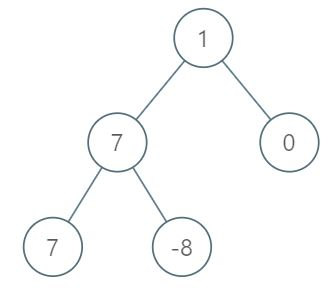``````输入：root = [1,7,0,7,-8,null,null]

``````

``````输入：root = [989,null,10250,98693,-89388,null,null,null,-32127]

``````

• 树中的节点数在 [1, 104]范围内
• -105 <= Node.val <= 105

BFS，一层一层遍历

BFS和DFS

# 【一天一题—Day10】593. 有效的正方形

## 二、题目

``````给定2D空间中四个点的坐标 p1, p2, p3 和 p4，如果这四个点构成一个正方形，则返回 true 。

``````

``````输入: p1 = [0,0], p2 = [1,1], p3 = [1,0], p4 = [0,1]

``````

``````输入：p1 = [0,0], p2 = [1,1], p3 = [1,0], p4 = [0,12]

``````

``````输入：p1 = [1,0], p2 = [-1,0], p3 = [0,1], p4 = [0,-1]

``````

• p1.length == p2.length == p3.length == p4.length == 2
• -104 <= xi, yi <= 104

# 【一天一题—Day9】1331. 数组序号转换

## 二、题目

``````给你一个整数数组 arr ，请你将数组中的每个元素替换为它们排序后的序号。

``````

``````输入：arr = [40,10,20,30]

``````

``````输入：arr = [100,100,100]

``````

``````输入：arr = [37,12,28,9,100,56,80,5,12]

``````

• 0 <= arr.length <= 105
• -109 <= arr[i] <= 109

# 【一天一题—Day6】919. 完全二叉树插入器

## 二、题目

``````完全二叉树 是每一层（除最后一层外）都是完全填充（即，节点数达到最大）的，并且所有的节点都尽可能地集中在左侧。

CBTInserter(TreeNode root) 使用头节点为 root 的给定树初始化该数据结构；
CBTInserter.insert(int v)  向树中插入一个值为 Node.val == val的新节点 TreeNode。使树保持完全二叉树的状态，并返回插入节点 TreeNode 的父节点的值；
CBTInserter.get_root() 将返回树的头节点。
``````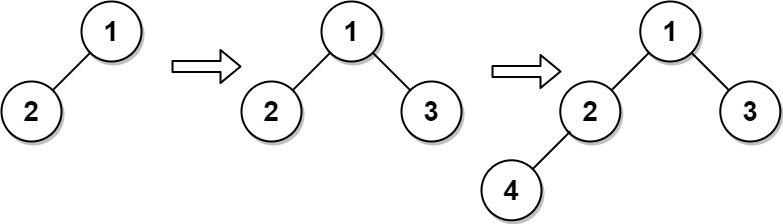``````输入
["CBTInserter", "insert", "insert", "get_root"]
[[[1, 2]], , , []]

[null, 1, 2, [1, 2, 3, 4]]

CBTInserter cBTInserter = new CBTInserter([1, 2]);
cBTInserter.insert(3);  // 返回 1
cBTInserter.insert(4);  // 返回 2
cBTInserter.get_root(); // 返回 [1, 2, 3, 4]
``````

• 树中节点数量范围为 [1, 1000]
• 0 <= Node.val <= 5000
• root 是完全二叉树
• 0 <= val <= 5000
• 每个测试用例最多调用 insert 和 get_root 操作 104 次

# 【一天一题—Day5】1184. 公交站间的距离

## 二、题目

``````环形公交路线上有 n 个站，按次序从 0 到 n - 1 进行编号。我们已知每一对相邻公交站之间的距离，distance[i] 表示编号为 i 的车站和编号为 (i + 1) % n 的车站之间的距离。

``````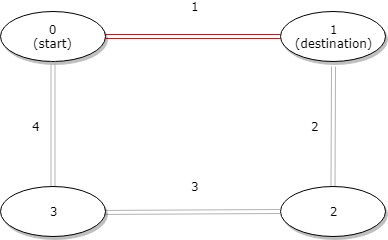``````输入：distance = [1,2,3,4], start = 0, destination = 1

``````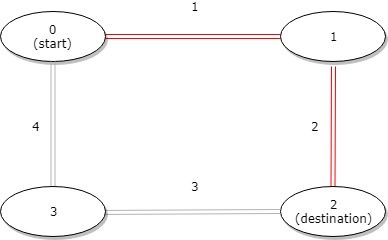``````输入：distance = [1,2,3,4], start = 0, destination = 2

``````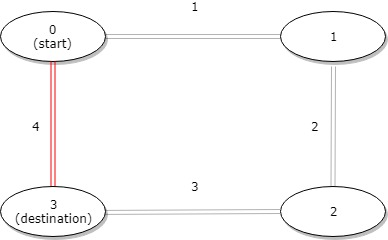• 1 <= n <= 10^4
• distance.length == n
• 0 <= start, destination < n
• 0 <= distance[i] <= 10^4

## 三、解答

### 2，官方解法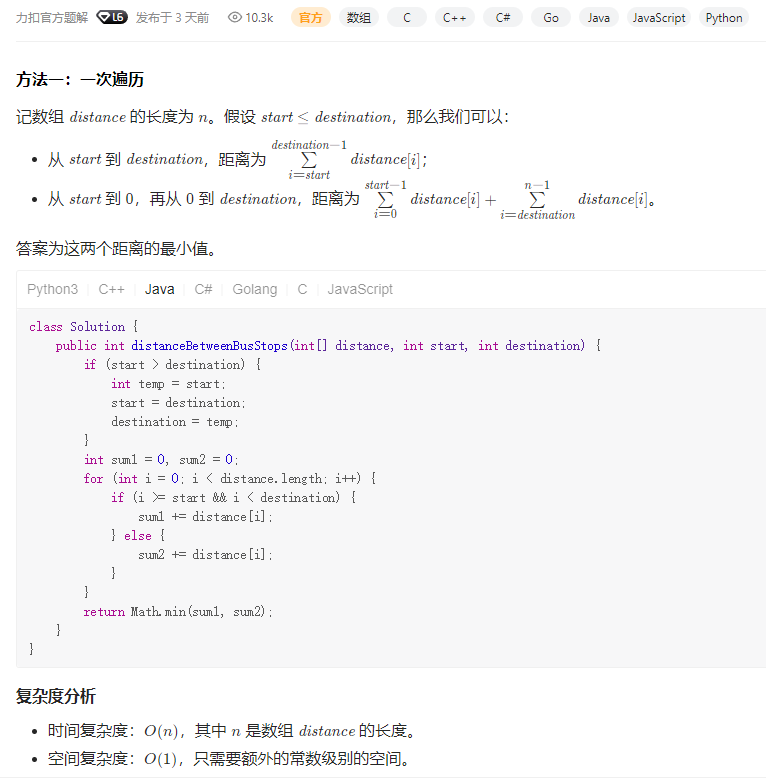# 【一天一题—Day4】剑指 Offer II 115. 重建序列

## 二、题目

``````给定一个长度为 n 的整数数组 nums ，其中 nums 是范围为 [1，n] 的整数的排列。

- 例如，对于 sequences = [[1,2],[1,3]] ，有两个最短的 超序列 ，[1,2,3] 和 [1,3,2] 。
- 而对于 sequences = [[1,2],[1,3],[1,2,3]] ，唯一可能的最短 超序列 是 [1,2,3] 。
[1,2,3,4] 是可能的超序列，但不是最短的。

``````

``````输入：nums = [1,2,3], sequences = [[1,2],[1,3]]

``````

``````输入：nums = [1,2,3], sequences = [[1,2]]

``````

``````输入：nums = [1,2,3], sequences = [[1,2],[1,3],[2,3]]

``````

• n == nums.length
• 1 <= n <= 104
• nums 是 [1, n] 范围内所有整数的排列
• 1 <= sequences.length <= 104
• 1 <= sequences[i].length <= 104
• 1 <= sum(sequences[i].length) <= 105
• 1 <= sequences[i][j] <= n
• sequences 的所有数组都是 唯一 的
• sequences[i] 是 nums 的一个子序列

## 三、解答

### 1，非官方解法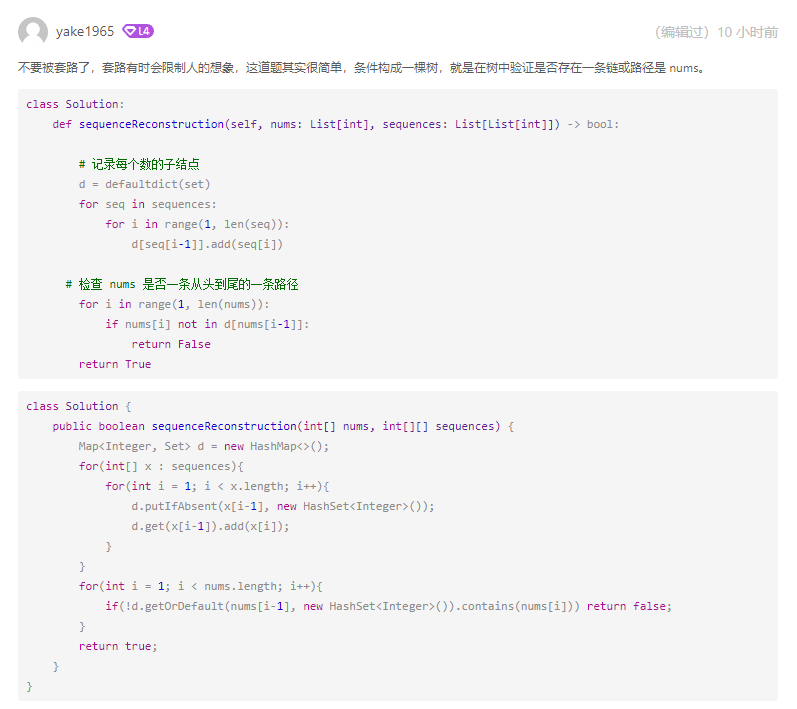# 【一天一题—Day2】814. 二叉树剪枝

## 一、前景提要## 二、题目

``````给你二叉树的根结点 root ，此外树的每个结点的值要么是 0 ，要么是 1 。

``````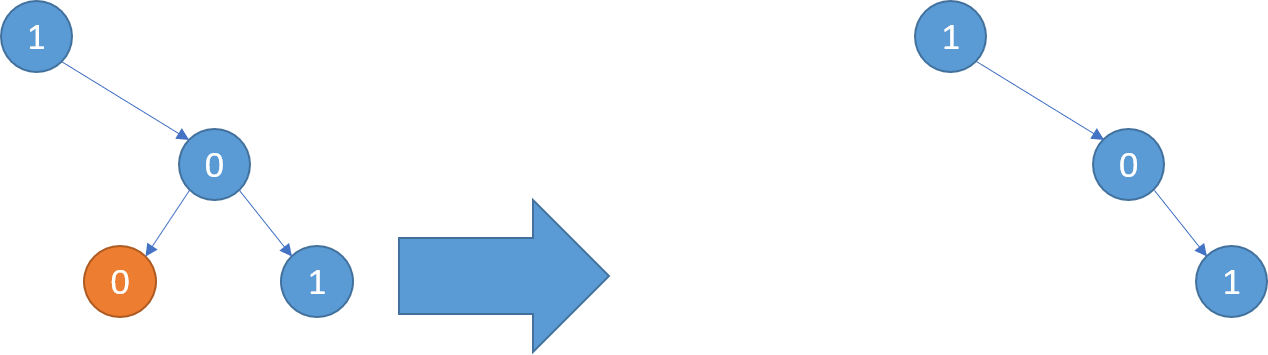``````输入：root = [1,null,0,0,1]

``````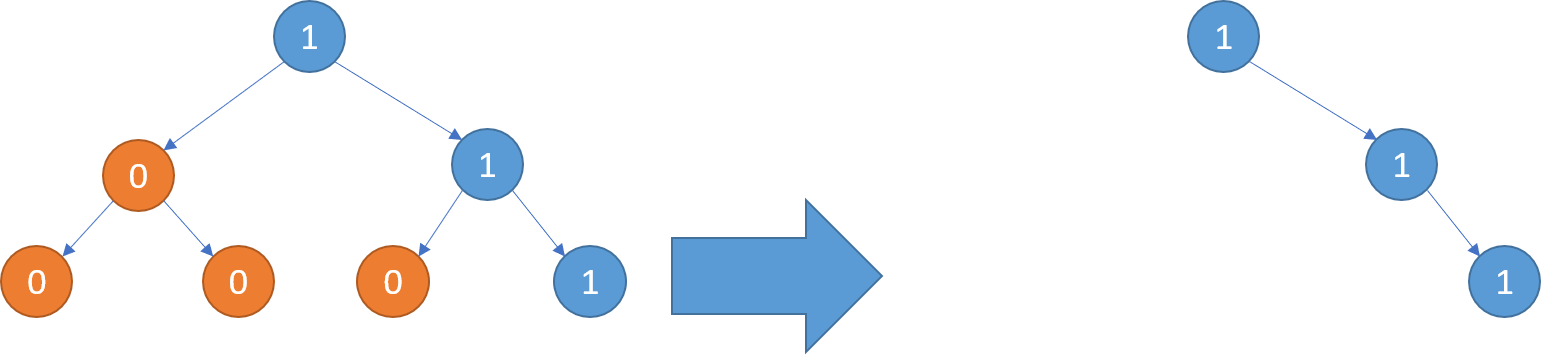``````输入：root = [1,0,1,0,0,0,1]

``````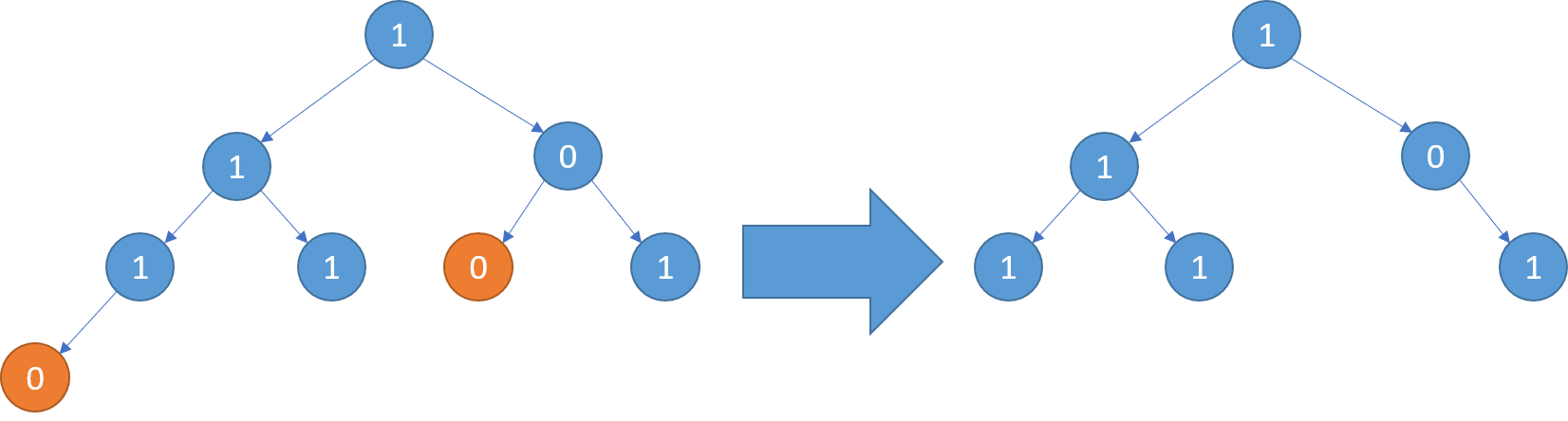``````输入：root = [1,1,0,1,1,0,1,0]

``````

• 树中节点的数目在范围 [1, 200] 内
• Node.val 为 0 或 1

## 三、解答

### 2，官方解法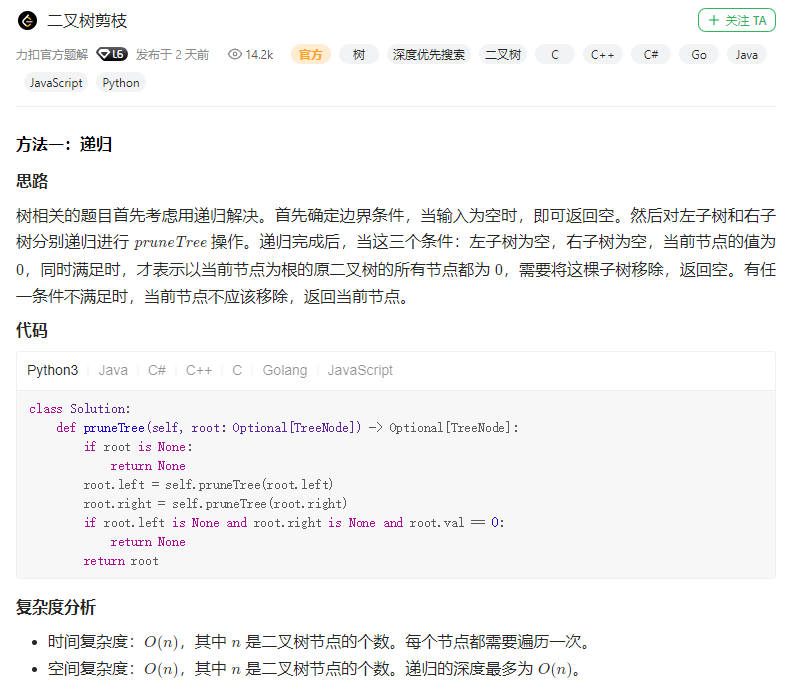# 【一天一题—Day1】1260. 二维网格迁移

## 一、前景提要## 二、题目

``````给你一个 m 行 n 列的二维网格 grid 和一个整数 k。你需要将 grid 迁移 k 次。

``````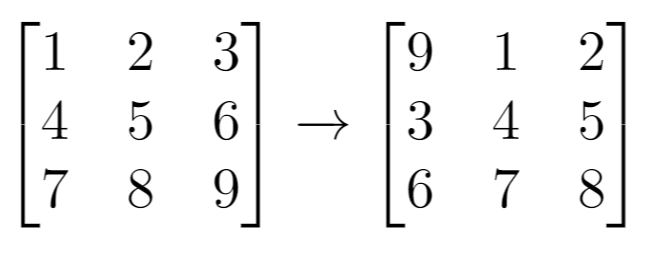``````输入：grid = [[1,2,3],[4,5,6],[7,8,9]], k = 1

``````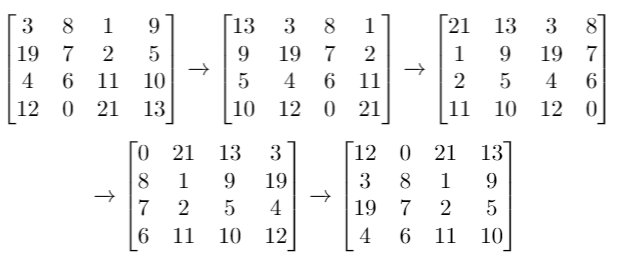``````输入：grid = [[3,8,1,9],[19,7,2,5],[4,6,11,10],[12,0,21,13]], k = 4

``````

``````输入：grid = [[1,2,3],[4,5,6],[7,8,9]], k = 9

``````

• m == grid.length
• n == grid[i].length
• 1 <= m <= 50
• 1 <= n <= 50
• -1000 <= grid[i][j] <= 1000
• 0 <= k <= 100

## 三、解答

### 1，暴力解法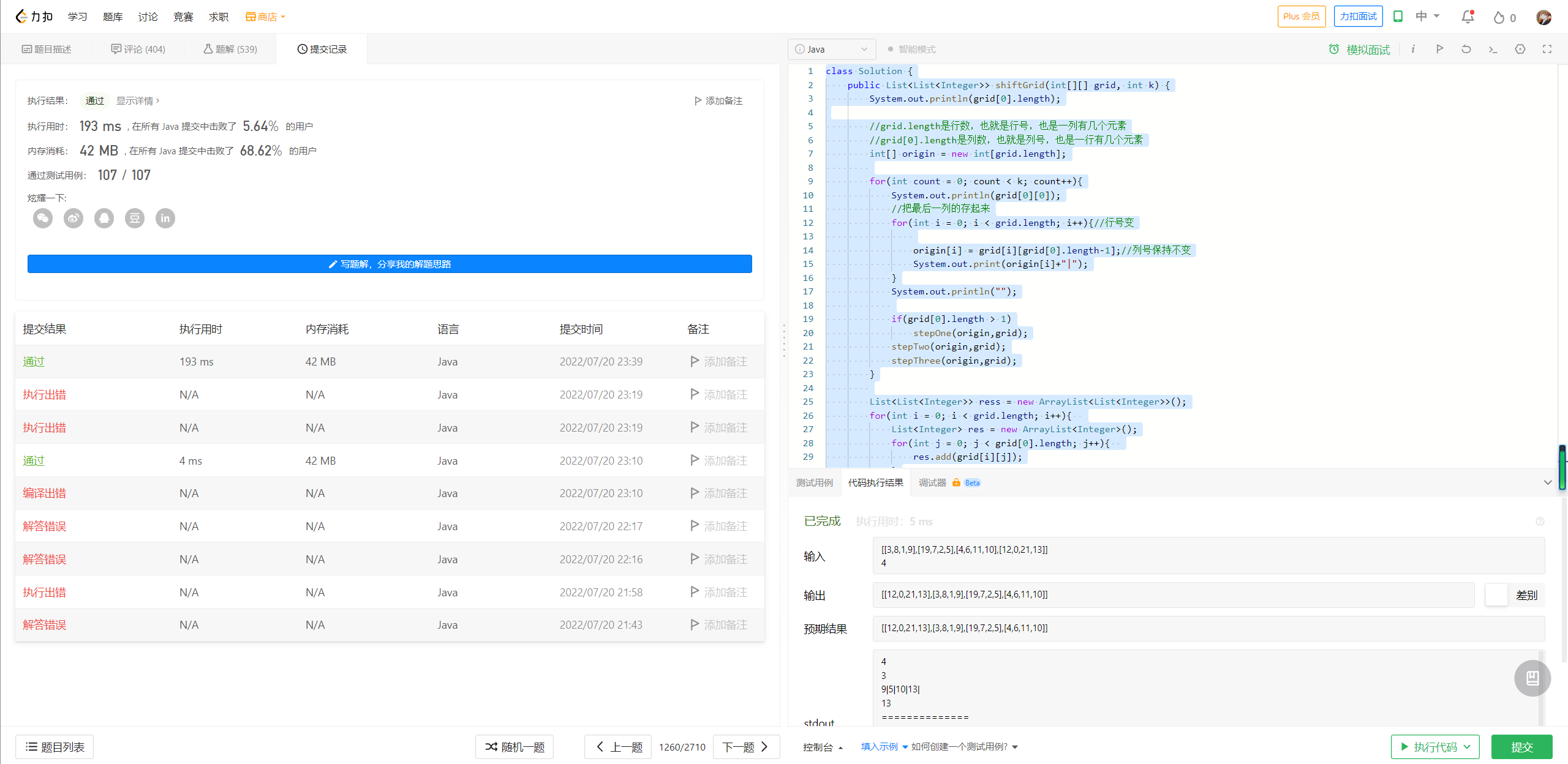### 2，官方解法

#### 然后循环右移

k次迁移后下标变成：`index1 = (index + k) % (m * n)`

#### 最后是还原

``````⌊x⌋：地板函数，或取整函数，表示小于 x 的最大整数；⌈x⌉：天花板函数，表示大于 x 的最小整数。
``````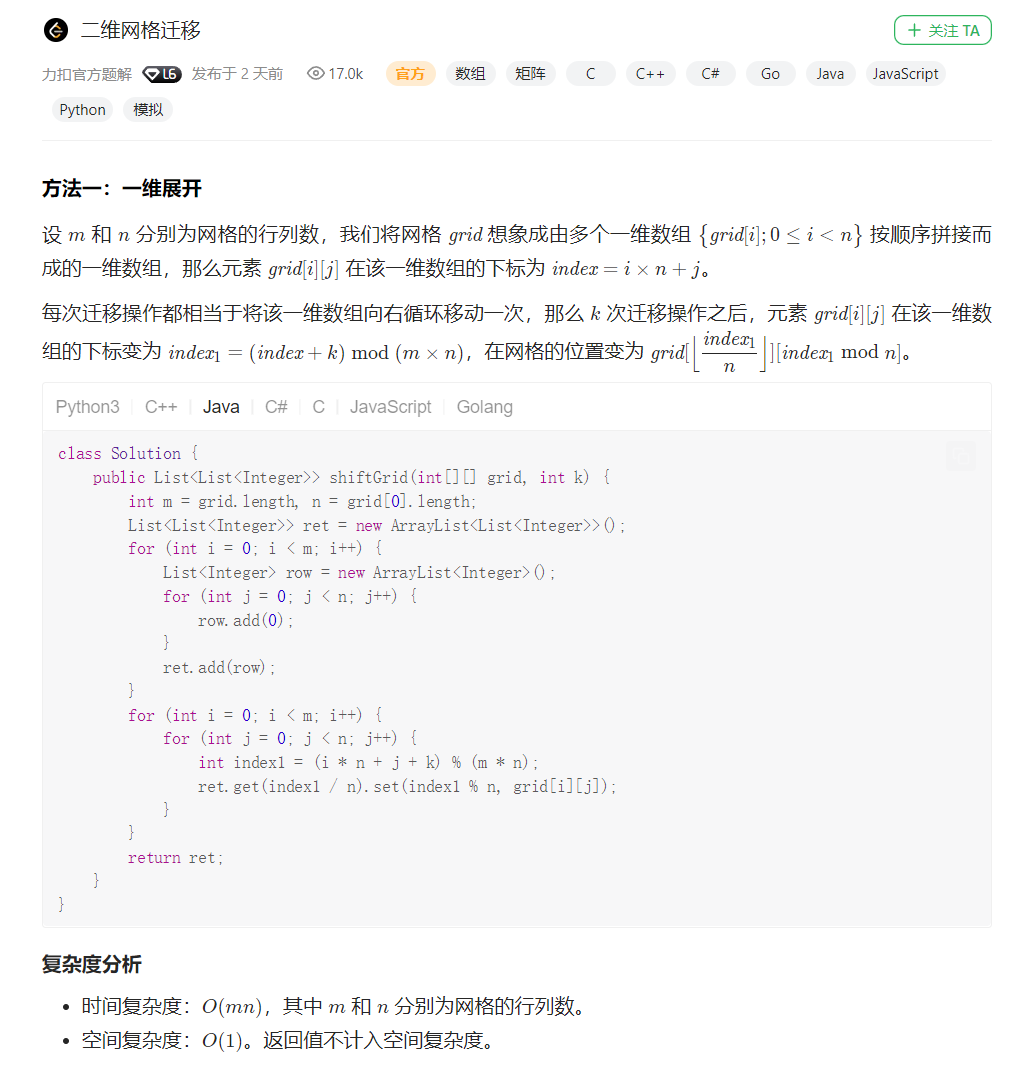【一天一题】力扣刷题日记
https://moechun.fun/2022/08/11/【一天一题】力扣刷题日记/

Knight Kilito

2022年8月11日

2023年3月23日